# Control System – Stability in Control System

Stability is the most important system specification.If the system is unstable, transient, and steady-state response will be meaningless. An unstable system can never be used for designing purpose by a system architect. This left us with some significant questions i.e  What happens if the system is unstable ? What makes any system unstable ? How do we ensure that a specific system is never unstable? Or can it even be ensured?

The way we achieve consistency in any electronics systems is by the means of the feedback. We can never achieve the desired output with an unstable system. Though there are many definitions for stability, we should keep our focus in mainly linear time invariant systems.

Stable System:

A system is said to be stable, if for bounded input the response of the system is bounded. In the absence of an input, a stable system approaches zero as time approaches infinity irrespective of the initial conditions. This condition is known as Bounded input Bounded output(BIBO).

Unstable System :

A system is said to be unstable if, for a bounded input, the system produces an output that goes on increasing( out of control) without any bounds and the designer has no control over it.

As a technical person, you might have worked with breadboards, IC’s and designed basic circuits. Moreover at some point, you might have surely burnt( just an assumption) any IC due to excessive heat. This burning off is a result of the system becoming unstable. In our daily life we can’t find any unstable system around us.

Marginal Stable System

Any system is marginally stable if the output of the system does not go down to zero like a stable system or does go on increasing (like an unstable system) . The output of a marginally stable system oscillates in a finite range.

How can we ensure that an electronic system is never unstable?

There is where control theory comes into picture, which helps in predicting the system stability. For the analysis of these systems we use poles and zeros. Poles and zeros are really some frequencies at which the system displays specific behaviour.

There are tools in the control theory, like Bode plot and root locus that help us theoretically understand the mechanisms by which we can ensure stability. Or what parameters, if changed can adversely affect stability.

Rather beating around the bush, we should now understand the mathematical definition for stability. According to which, a system is stable if and only if all the ‘Closed Loop Poles’ of the system lie on the left half plane (LHP) .

Note : The s-plane is divided into three main parts i.e the right half plane (RHP) , imaginary axis (jω axis)imaginary axis (jω axis) and the left half plane (LHP) .

We have to now introduce one new term here, i.e ‘Characteristic equation’. Let us first understand what it is:

We already know the closed loop transfer function  :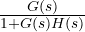So now the characteristic equation of any closed loop transfer function (CLTF) is given by equating the denominator of CLTF to 0.

i.e         1 + G(s)H(s) = 0

Let’s look one example, for one system ,

G(s) =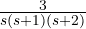and    H(s) =  1

Then its characteristic equation (CLTF denominator = 0)  =   1 + G(s)H(s) = 0

Thus, on solving

s(s + 1) (s + 2) + 3 = 0

On solving we will get roots as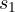= -2.672  ,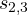= -0.164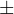j 1.047 ,

All poles lie on LHP.

Note:  you may wonder, why this is called the characteristic equation??

So these are called characteristic equation of their respective system as they give us the character (whether stable or unstable) of the system.

Last but not the least, finding roots of any characteristic equation is easy, if the maximum power of ‘s’ in the denominator is 2 or at most 3. But think if the power is very large like 6 or may be 8, then what??……See you in my next post with method to analyse such characteristic equation.